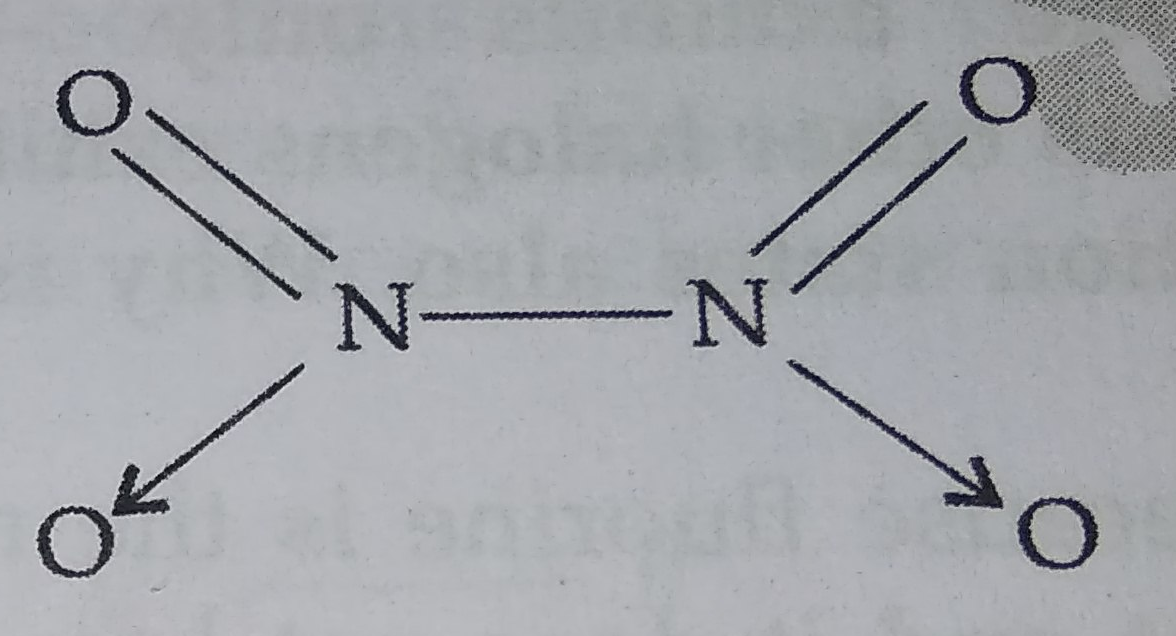Why does $N{O}_{2}$ dimerise?

Concept Videos :-

#2 | 15th Group: Properties: II
#3 | 15th Group: Ammonia

Concept Questions :-

group 15 ,preparation and properties

$N{O}_{2}$ contains 7+2$×$8 i.e., 23 odd electrons. In the valence shell N has seven electrons and hence less stable. To acquire stability it dimerizes to form ${N}_{2}{O}_{4}$Difficulty Level:

• 91%
• 0%